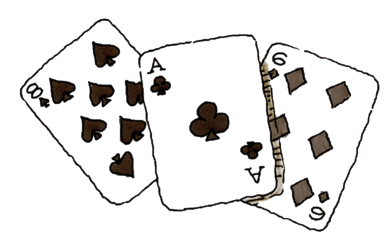### Home > CCG > Chapter 1 > Lesson 1.2.6 > Problem1-107

1-107.

What is the probability of drawing each of the following cards from a standard playing deck? See the entry “playing cards” in the glossary to learn what playing cards are included in a deck.

Review the Math Notes box in Lesson 1.2.1.

Recall that there are $52$ cards in a deck.There are four suits: clubs, diamonds, hearts, and spades.
Each suit has 13 cards: $A, 2, 3, 4, 5, 6, 7, 8, 9, 10, J, Q, K$

1. $P(\text{Jack})$

$\large \frac{\text{Number of Jacks}}{52\ {\text{cards}}}$

$\frac{4}{52}$

2. $P(\text{spade})$

3. $P(\text{Jack of spades})$

4. $P(\text{not spade})$